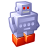# New to Qlik Sense

If you’re new to Qlik Sense, start with this Discussion Board and get up-to-speed quickly.

Announcements
QlikWorld May 10-12, Online and Free! REGISTER NOW
cancel
Showing results for
Did you mean:Contributor

## Previous Month expresion

Hello,

I have used and following expression to sum the values for current month which works fine:

SUM({\$<[Date]={'>=\$(=MonthStart(Today()))<=\$(=MonthEnd(Today()))'}>}[Sales Actual])

I need to find separately values for previous month trying below expression, but it doesn't work:

SUM({\$<[Date]={'>=\$(=MonthStart(Today()),-1))<=\$(=MonthEnd(Today()),-1))'}>}[Sales Actual])

rgds

Jacek

5 RepliesSpecialist II

Hi

Suppress the comma before -1 lire this :

SUM({\$<[Date]={'>=\$(=MonthStart(Today())-1))<=\$(=MonthEnd(Today())-1))'}>}[Sales Actual])Creator II

extra ) in there after today(), the ,-1 is part of the monthstart arguments

SUM({\$<[Date]={'>=\$(=MonthStart(Today(),-1))<=\$(=MonthEnd(Today(),-1))'}>}[Sales Actual])Master II

Use Master Calendar and it's Flags to save the pain for using complex Set analysis in front end.

Flags are fairly easy to use.

Master Calendar Script

Sum({< LastMonthFlag = {1}>}[Sales Actual])Champion III

Try:

SUM({\$<[Date]={'>=\$(=MonthStart(Today(),-1))<=\$(=MonthEnd(Today(),-1))'}>}[Sales Actual])Partner

You can also try